# Using the COUNTIF and COUNTIFS functions and Remove Duplicates in Excel 2007 and Excel 2010

## How to use Remove Duplicates, COUNTIFS and COUNTIF in Excel 2007 and Excel 2010

Hi and welcome to my latest hub on Excel. Today, we are going to look at the COUNTIF and COUNTIFS functions, along with Remove Duplicates.

• COUNTIF allows you to count the number of cells within a range that contain what you are asking Excel to look for. So for example, how many cells in the range A1 to B53 contain the word Paul or end with the word Jones?
• COUNTIFS expands on what the COUNTIF function does and allows you to use multiple criteria. For example, you can ask Excel to count the number of times Paul Jones appears in a range, or the number of A Jones that appear.

Note: Both COUNTIF and COUNTIFS allow you to use the wildcards * and ? within their formulas which we will cover here in depth.

• Finally, we will look at using Remove Duplicates in Excel. This allows you to take a large amount of data and remove all duplicated entries, leaving you with a list of unique items. This is a particularly useful tool if you are trying to tidy up or summarise a large amount of data.

I have a hub that covers the SUMIF and SUMIFS functions which operate in a very similar manner to COUNTIF and COUNTIFS, except they allow you to sum cells containing what you are asking Excel to look for rather than to count them. That hub can be found here:

http://robbiecwilson.hubpages.com/hub/How-to-use-the-SUMIF-and-SUMIFS-functions-formulas-in-Excel-2007-and-Excel-2010-with-examples

## Using COUNTIF in Excel 2007 and Excel 2010

In my first example, I have a small team of salesmen. At the end of every day, we record if they have made a sale or not. Over the period of a week, I would like to know how many times each salesman has sold. We start off with our raw data which looks like this: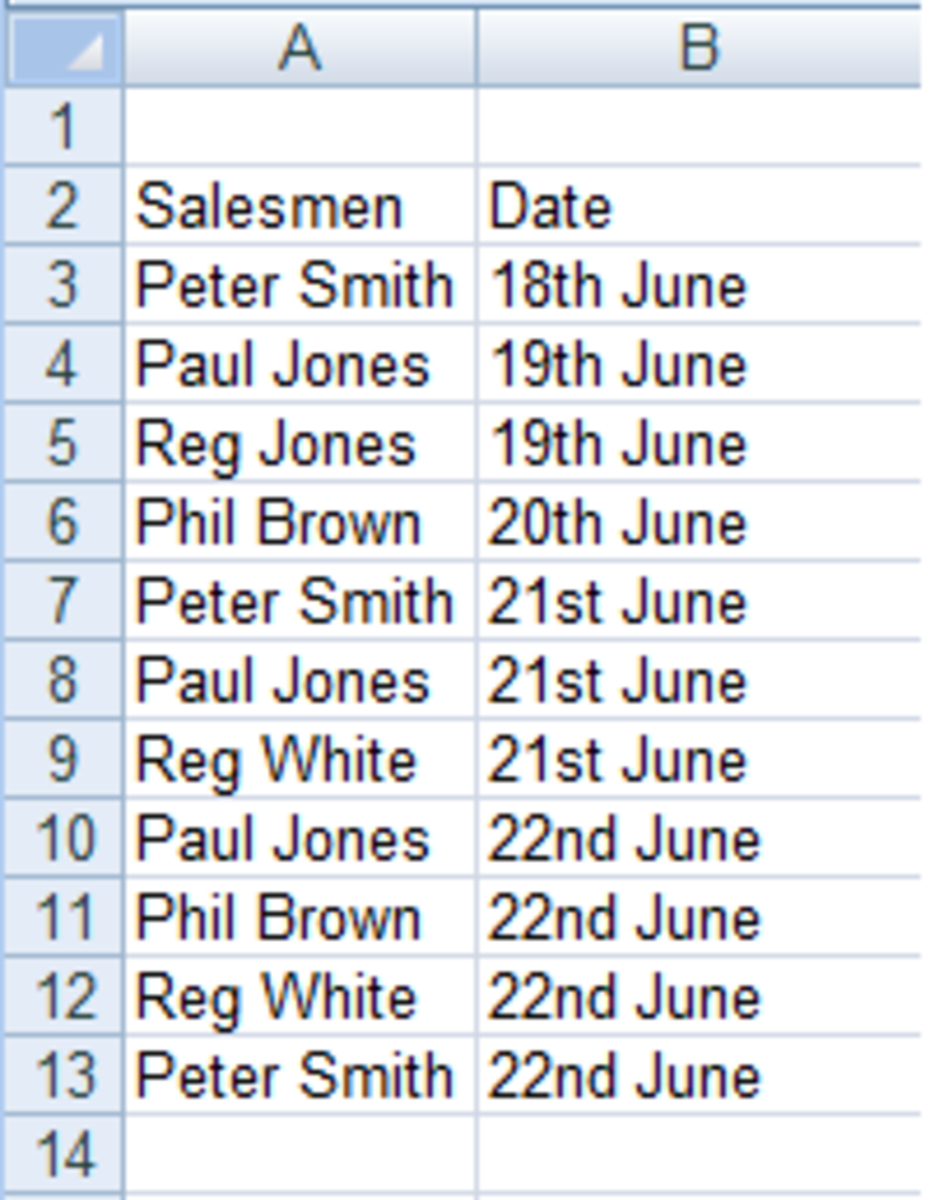Raw data from our salesmen in Excel 2007 and Excel 2010.http://robbiecwilson.hubpages.com/

The syntax of the COUNTIF formula is made up of two parts:

• The first is the range you are asking Excel to look in (in this example, A3:A13)
• The second is what you are asking Excel to count (Peter Smith)

Note: There are two ways of writing the COUNTIF formula, the first asks Excel to look for the contents of A3 and the second is to look for “Paul Smith”. Either way is fine, the second is easier to read.

Here is our finished formula:

=COUNTIF(A3:A13,A3)

OR

=COUNTIF(A3:A13,"Peter Smith")

We want to now create a table that shows the sales figures for our salesmen for the week ending the 22nd of June. We create a table containing the names of our salesmen and then we use COUNTIF to create formulas for each member of the team. You can see the completed table below: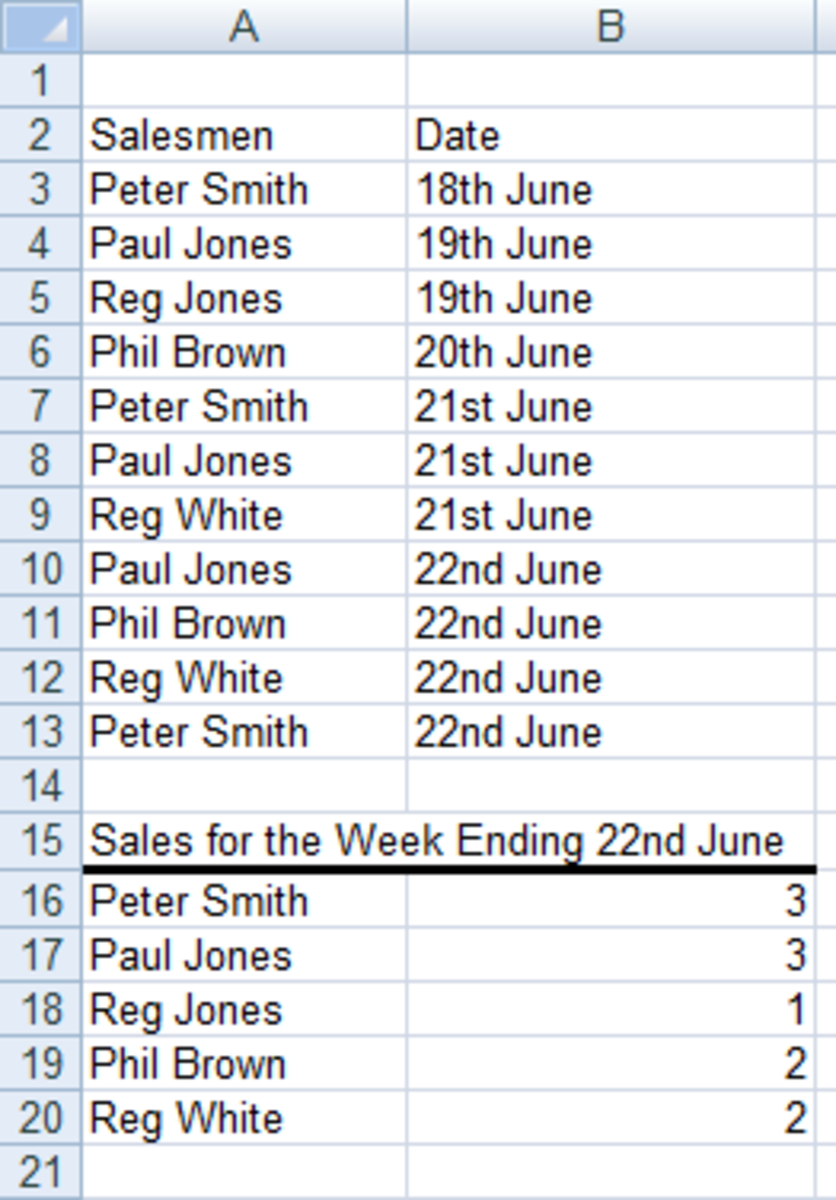Table created using data from COUNTIF formulas created in Excel 2007 and Excel 2010.http://robbiecwilson.hubpages.com/

To create our table, we use the following formula for Peter Smith:

=COUNTIF(\$A\$3:\$A\$13,A16)

Scroll to Continue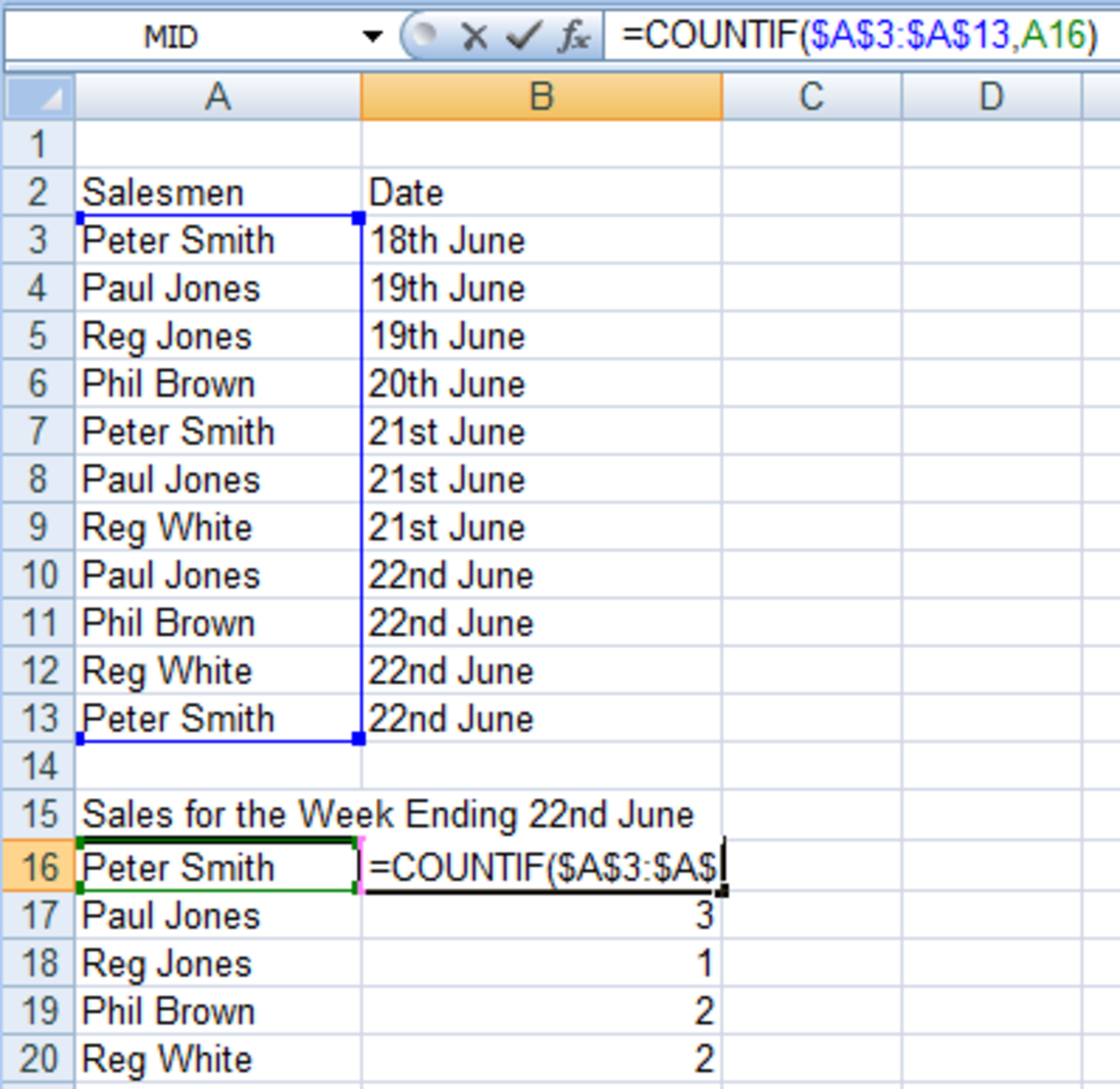Example of a COUNTIF formula created in Excel 2007 and Excel 2010.http://robbiecwilson.hubpages.com/

We then copy the formula into B17 to B20 to complete the formulas for the other team members

Note: We use the \$ in our formula to ensure that Excel uses the exact same range for each formula.

Note: We use the cells in the table rather than the range so that we can use the same formulas from week to week as we can be sure that these will not change. If we used =COUNTIF(A3:A13,A3) and Peter Smith was not the first salesmen to sell next week then we would be counting another salesman’s figures and giving him credit for them.

## Using COUNTIFS to count more than one criterion in Excel 2007 and Excel 2010

Over time, the number of salesmen in our company has increased and they have been divided into two teams. To make matters more complicated, we have a Peter Smith in both teams!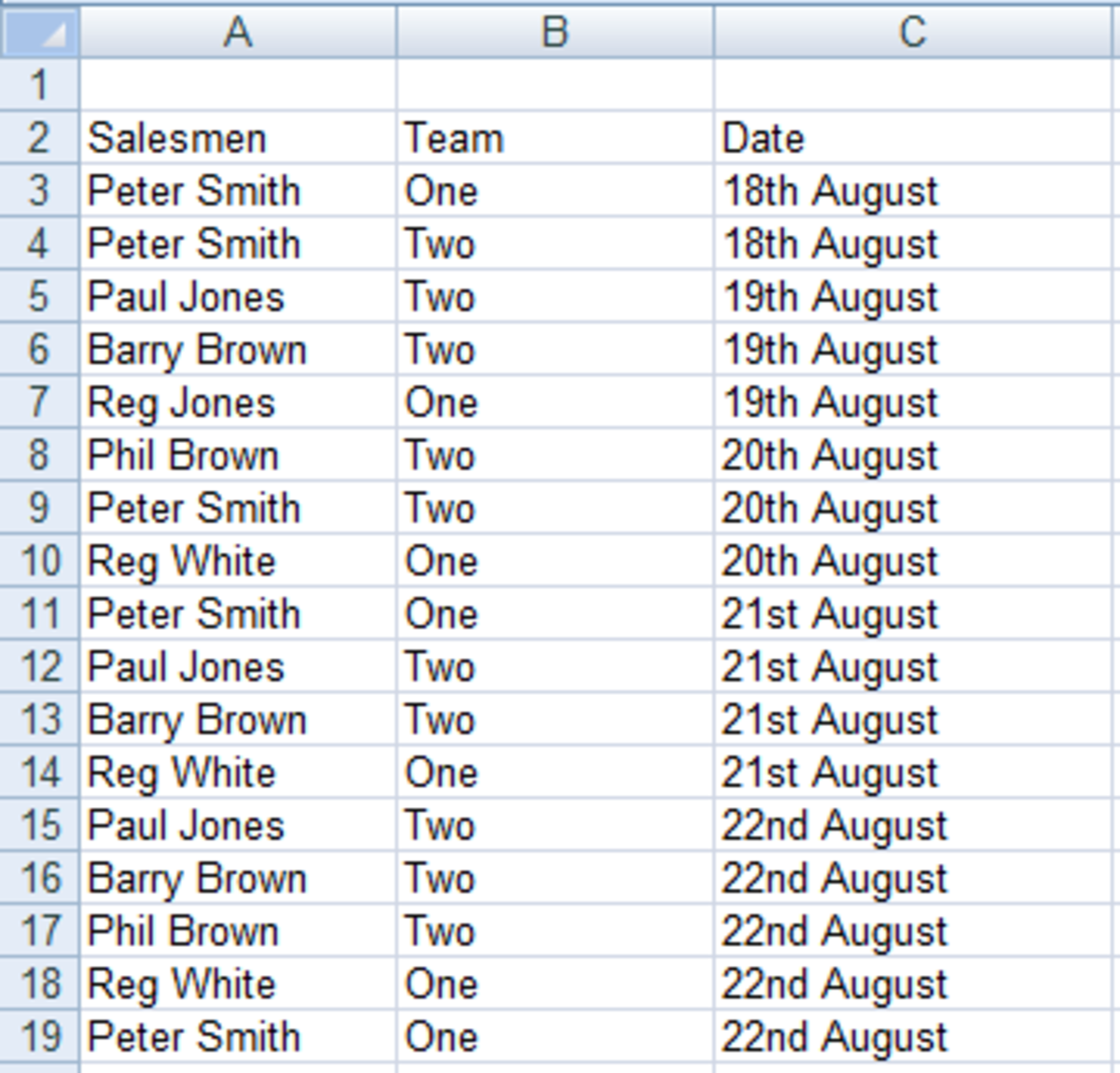Raw data showing the extended sales teams in Excel 2007 and Excel 2010.http://robbiecwilson.hubpages.com/

COUNTIFS works in a very similar way to COUNTIF, but it allows you to add a second (or third, fourth, fifth etc.) criteria. So for Peter Smith from Team One the formula is as follows:

=COUNTIFS(\$A\$3:\$A\$19,A23,\$B\$3:\$B\$19,B23)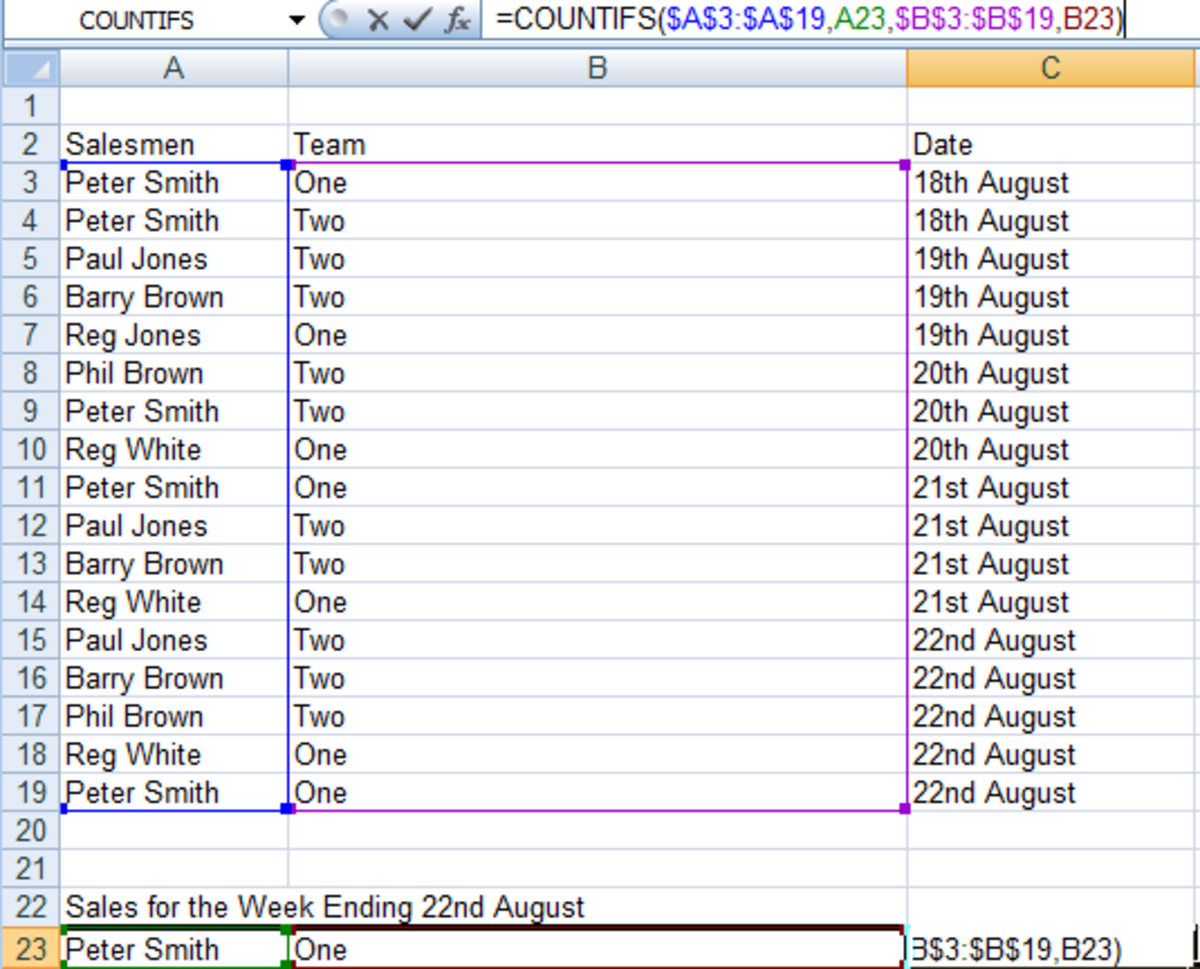Example of a COUNTIFS formula created in Excel 2007 and Excel 2010.http://robbiecwilson.hubpages.com/

You can also use the Function Library to construct the formula. To access this:

• Click on the Formulas tab
• Select More Functions and then Statistical
• Choose COUNTIFS and complete the dialogue boxes as below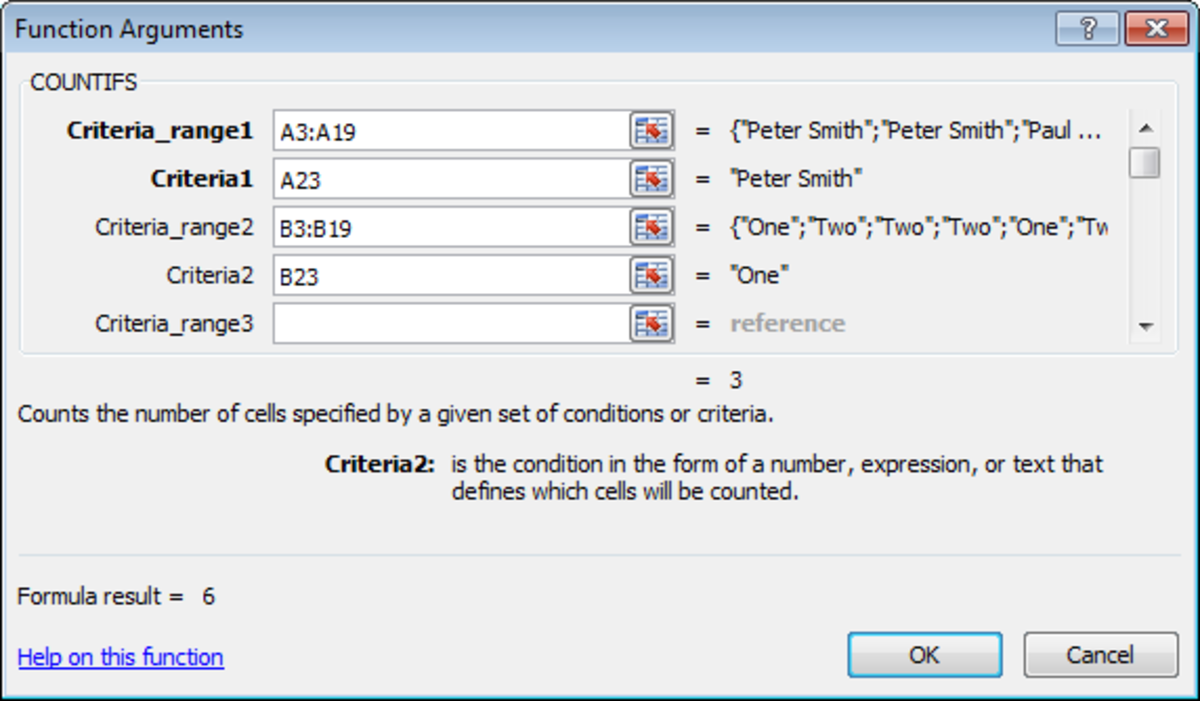Using the Function Library to create a COUNTIFS formula in Excel 2007 and Excel 2010.http://robbiecwilson.hubpages.com/

If you would like to know more about the Function Library and using it to build formulas, I have a hub that shows how to best use this very powerful function. Not only can you pick from a list as we did, but you can type in what you are trying to achieve and ask Excel to advise you on which formula to use which is very handy!

http://robbiecwilson.hubpages.com/hub/How-to-create-a-formula-in-Excel-using-the-Function-Library-in-Excel-2007-and-Excel-2010

• We can now copy the formula to each of salesmen and we end up with our new table for the week ending the 22nd of August: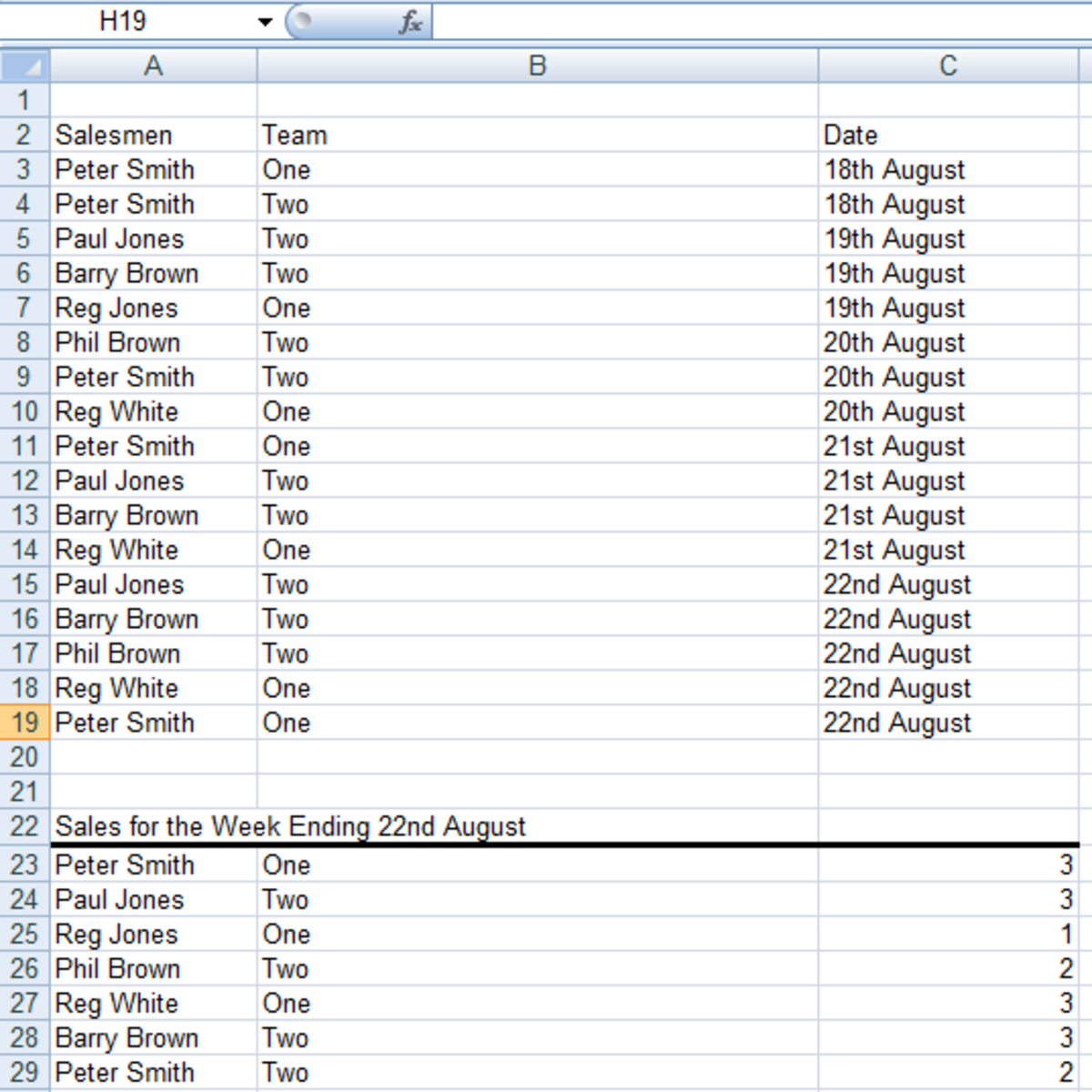Table created using a COUNTIFS formula in Excel 2007 and Excel 2010.http://robbiecwilson.hubpages.com/

## Using Wildcard characters with COUNTIF and COUNTIFS in Excel 2007 and Excel 2010

There are two wildcards that you can use with both functions in formulas:

The ? is used to replace a single character. So from our first example:

• If you gave Excel the following formula =COUNTIF(\$B\$3:\$B\$19,"??e") it will count all the cells that have e as the third character which is 7.

The * wildcard is best used when you don’t have the same number of characters before or after the text you are asking Excel to look for. So for example:

To count all cells that contain text that starts with a P: =COUNTIF(\$A\$3:\$A\$19,"P*")

To ask Excel to count everything ending with Jones: =COUNTIF(\$A\$3:\$A\$19,"*Jones")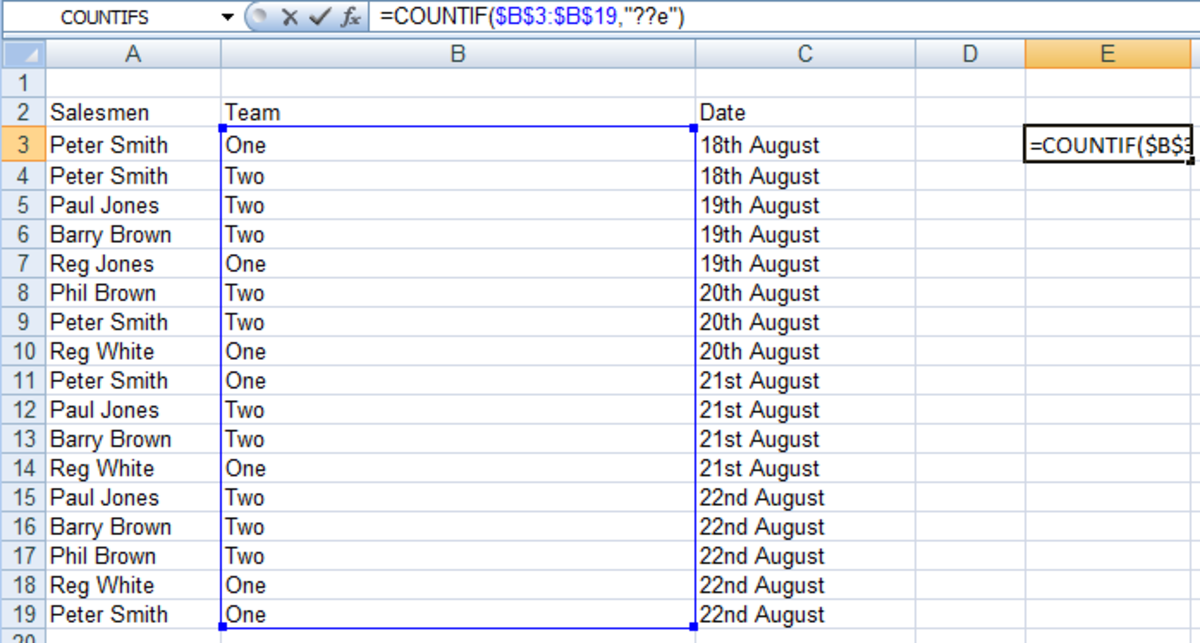An example of how to use wildcards in a COUNTIF formula in Excel 2007 and Excel 2010.http://robbiecwilson.hubpages.com/

## Using Excel 2007 and Excel 2010 to remove duplicates from your workbook

Particularly in very large Excel workbooks, duplication is common place and very difficult to spot. We can use the Remove Duplicates button to create unique lists of data stored in your spreadsheets. If we go back to our example of our team of salesmen, to create the table of salesmen, rather than typing them out manually we can create a list of unique names and copy them to the new table. You can additionally specify which columns are to be checked.

• First, we need to select the data we want Excel to check for duplicates, so in our example above we select A3 to A19 (we will worry about sorting into teams later)
• Click on the Remove Duplicates button on the Data tab in the Data Tools group
• Excel can see that there is data in column B so it asks us to Expand the selection or Continue with the current selection. In this example we will continue with just column A
• Excel then reports on how many items were removed

Leaving us with the following list: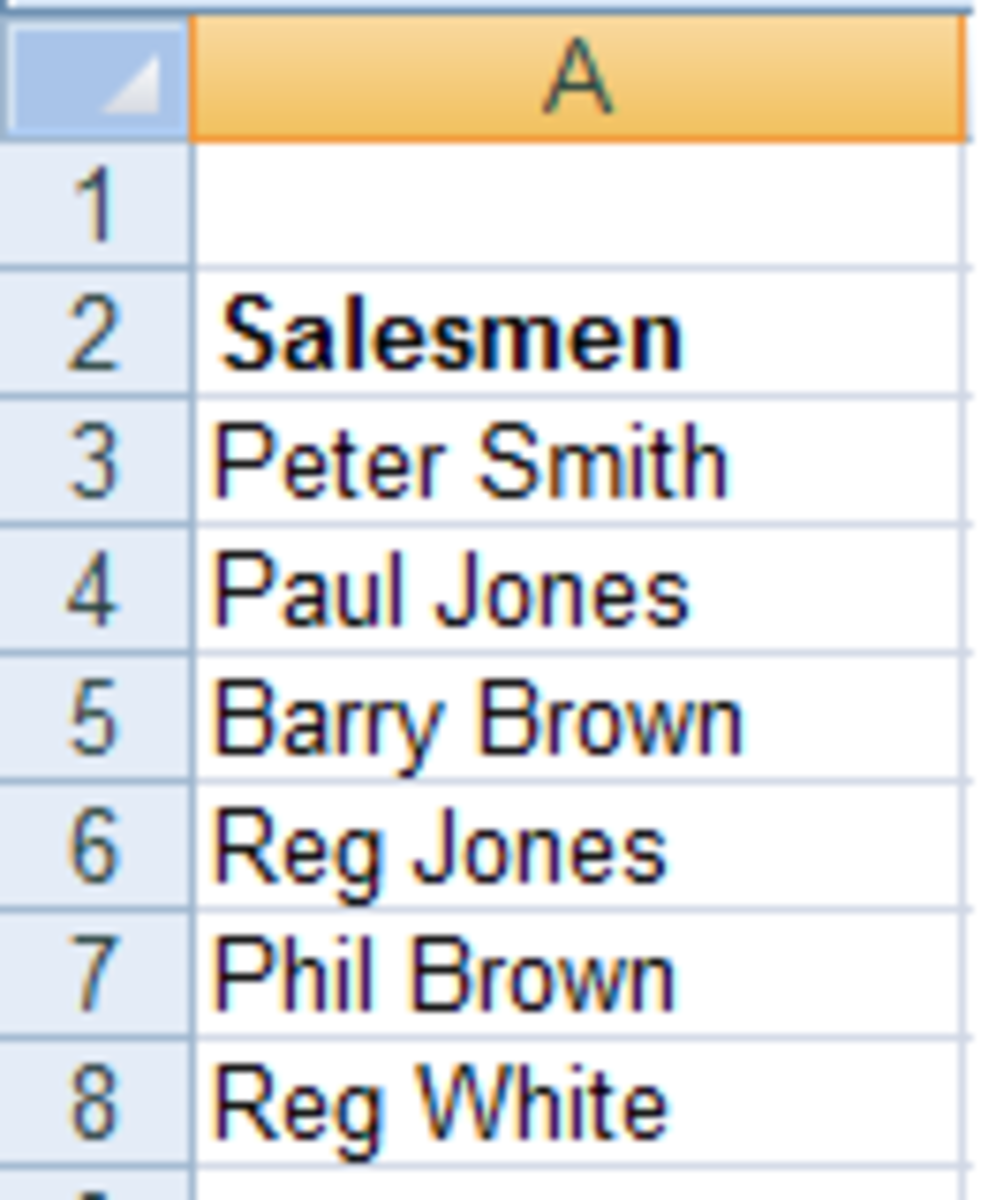List of unique items created using Remove Duplicates in Excel 2007 and Excel 2010.http://robbiecwilson.hubpages.com/

If we repeat the above process, but selecting A3 to B19, we get our salesmen and the team that are in as well: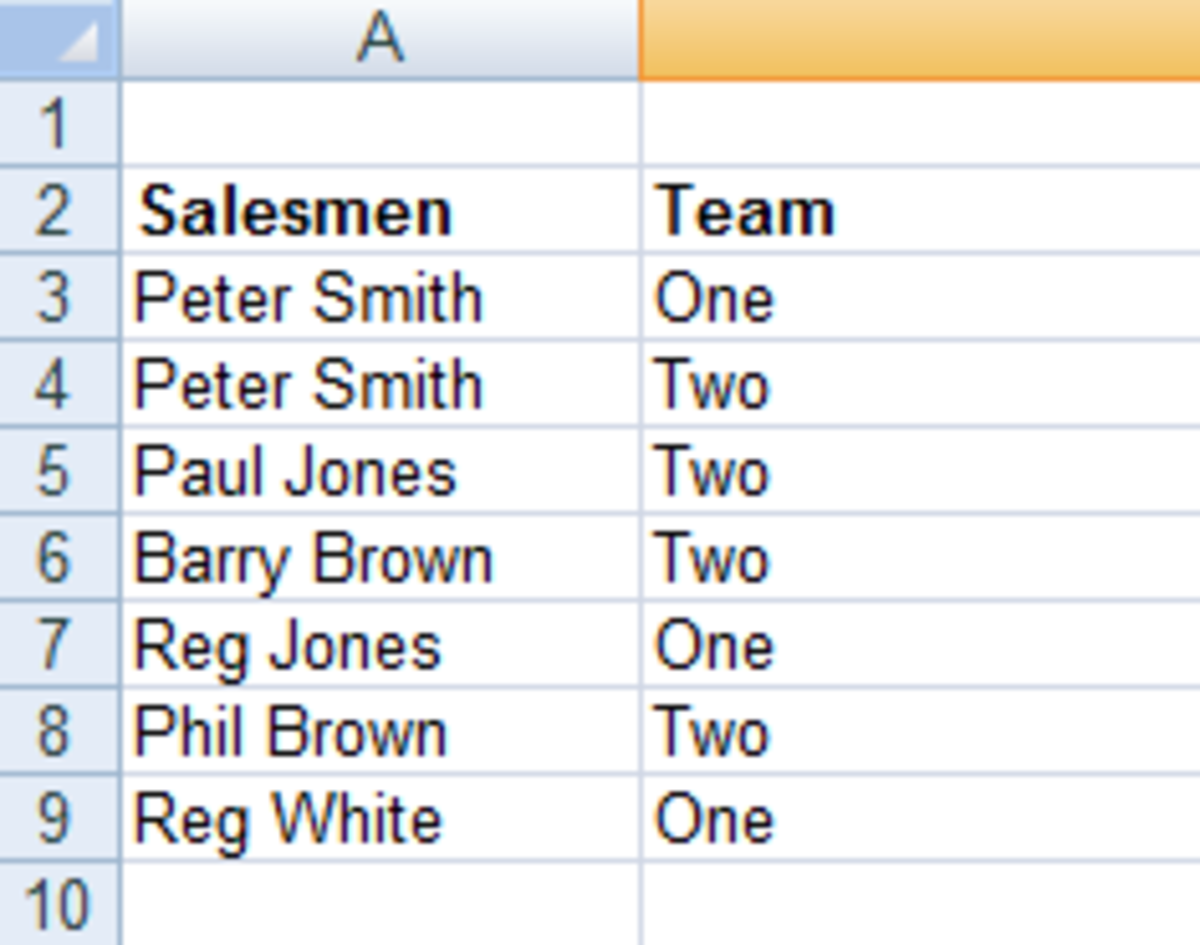List created by Remove Duplicates using an expanded selection of two columns in Excel 2007 and Excel 2010.http://robbiecwilson.hubpages.com/

## Conclusion

In today’s hub, I introduced two very useful functions that allow you to count instances of something you are interested in within a specific range of cells:

• The COUNTIF function allows you to count the number of times something occurs within a particular range of cells for example, how many times Peter Smith is found in cells A3 to A19
• COUNTIFS allows you to expand this to multiple criteria, so we can count the number of instances of Peter Smith in team One occurs in the range A3 to B19
• We also examined how to use the ? and * wildcards in your COUNTIF and COUNTIFS formulas
• Finally, we looked at the Remove Duplicates button which allows you to easily and quickly summarise or tidy up data by removing everything in a range of cells that occurs more than once. You can also expand the selection to include multiple columns if your data requires it

Thanks for reading my hub on using the COUNTIF and COUNTIFS functions as well as Remove Duplicates. I hope you have enjoyed reading my hub as much as I have enjoyed writing it and that you found it useful and informative. Please feel free to leave a comment below.